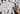# Solve Room Maze With Table Q(0) RL Method

In this post we provide a Python solution for the room maze defined by mnemstudio.org. The solution will be based solely on Python and numpy using a tabular Q(0) reinforcement learning algorithm, also known as Q learning.

### Problem description

The link above already provides a good outline of the problem, so let us keep things short:

The maze consists of six rooms, some of them are connected by doors others have no connection. The agent will be placed randomly in one of the rooms and has to find it's way until the agent reaches room number 5, which is defined as outside. The other rooms are labeled 0 - 4. The maze provides two ways to leave the building, either through room 1 or through room 4. Both of them provide an exit.

### Environment

Given the room maze a reward matrix $R$ can be derived. It contains the reward given a state (row), i.e. to be in room $S_i$ where $i$ is the room number, and choosing an action (column) which let the agent go to room $A_j$ where $j$ is the new room number. The reward is then given by $R(i, j)$.

      A0  A1  A2  A3  A4  A5
S0 [[ -1  -1  -1  -1   0  -1]
S1  [ -1  -1  -1   0  -1 100]
S2  [ -1  -1  -1   0  -1  -1]
S3  [ -1   0   0  -1   0  -1]
S4  [  0  -1  -1   0  -1 100]
S5  [ -1   0  -1  -1   0 100]]

Example, say we are in room 3 ($S_3$) and the user chooses to go to room 1 ($A_1$). The reward of this move is described by position $(3, 1)$,in the rewards table. It gives a reward of $0$, because room 1 is not the terminal state. Also notice that the environment tells us which actions are possible given a state. Possible actions are those columns whose value is larger than $-1$. Terminal states have a reward of $100$, whereas all other states have a reward of $0$.

Note that $R(5,5)$ is defined and yields a reward of $100$, whereas $R(5,1)$ and $R(5,4)$ are defined as $0$. Since we explicitly allow the agent to leave terminal state if he has been started in room 5, we also give him the opportunity to stay in room 5, which is different to all other rooms. One could also re-write the problem to never let the agent start in a terminal state, which is how problems are often defined elsewhere.

### Problem classification

The problem is discrete and episodic. It satisfies the Markov property, because the future depends only on the current state and action, but not on the past.

### Algorithm

The following pseudocode has been taken from Sutton and Barto (2017). Its inner loops have been adjusted, to run at least once - even if the initial state is a terminal state.

#### SARSA

This pseudocode describes the SARSA(0) (state, action, reward, (next) state, (next) action) learning method.

Output: action value function $Q$
initialize $Q$ arbitrarily, e.g. to 0 for all states, set action value for terminal states as 0
for each episode do
....initialize state $s$
....forever do
........a $\leftarrow$ actions for $s$ derived by $Q$ ($\epsilon$-greedy)
........take action $a$, observe $r, s'$
........$a' \leftarrow$ actions for $s'$ derived by $Q$ ($\epsilon$-greedy)
........$Q(s,a) \leftarrow Q(s,a) + \alpha [r + \gamma Q(s', a') - Q(s,a) ]$
........$s \leftarrow s'$
........if state $s$ is terminal state do
............break
........end
....end
end

#### Tabular Q(0) Method

Output: action value function $Q$
initialize $Q$ aribitrarily, e.g. to 0 for all states, set action value for terminal states as 0
for each episode do
....initialize state $s$
....forever do
........a $\leftarrow$ actions for $s$ derived by $Q$ ($\epsilon$-greedy)
........take action $a$, observe $r, s'$
........$Q(s,a) \leftarrow Q(s,a) + \alpha [r + \gamma \max\nolimits_{a'} Q(s',a') - Q(s,a) ]$
........$s \leftarrow s'$
........if state $s$ is terminal state do
............break
........end
....end
end

### Python implementation

The full solution it too lengthy to show it here. Instead we provide it as a jupyter notebook.

### Results

Both algorithms converge and are able to solve the maze. The is what the final Q table (normalized) looks like.

      A0    A1    A2    A3    A4    A5
S0 [[ 0.    0.    0.    0.    0.8   0.  ]
S1  [ 0.    0.    0.    0.64  0.    1.  ]
S2  [ 0.    0.    0.    0.64  0.    0.  ]
S3  [ 0.    0.8   0.51  0.    0.8   0.  ]
S4  [ 0.64  0.    0.    0.64  0.    1.  ]
S5  [ 0.    0.8   0.    0.    0.8   1.  ]]

Using this table as the policy for the agent, we can place the agent somewhere in the maze, say room 2 and just follow the ruls in the table. From room 2 he choose the best action available, which is $A_3 = 0.64 \rightarrow S_3$. From there he can choose either $A_1$ or $A_4$, which are both similar in their Q values. Assume he picks $A_1 = 0.8 \rightarrow S_1$. From $S_1$ the best action is $A_5 = 1.0$. This brings the agent in room 5, which is the terminal room and finishes the episode.

So the full chain is then $S_2 \rightarrow A_3 \rightarrow S_3 \rightarrow A_1 \rightarrow S_1 \rightarrow A_5 \rightarrow S_5$

Problem solved 😊